Homework Help Question & Answers

# (4) (12 points)For the differential equation: Compute the recursion formula for the coefficients of the power series solution centered at o 0 and use it to compute the first three nonzero terms of...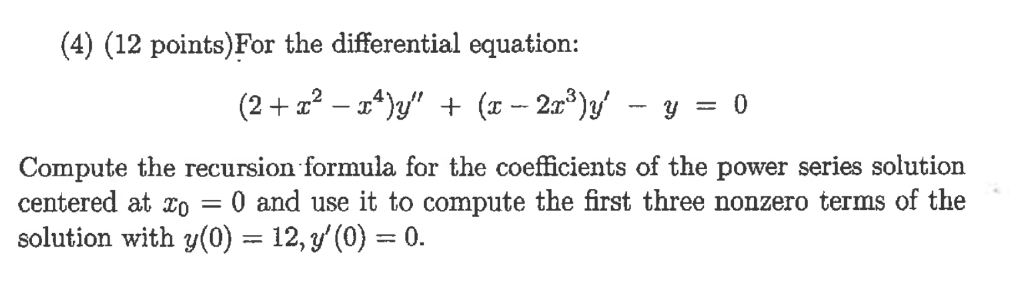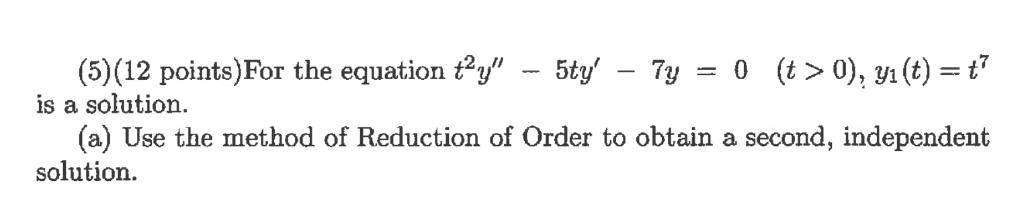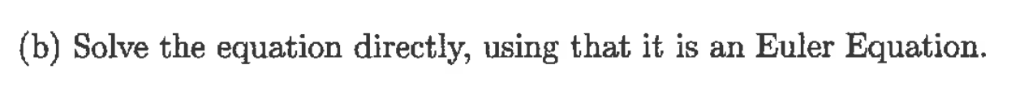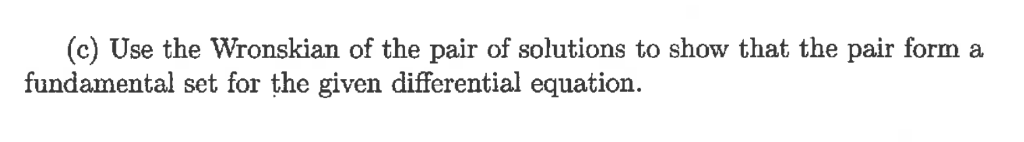(4) (12 points)For the differential equation: Compute the recursion formula for the coefficients of the power series solution centered at o 0 and use it to compute the first three nonzero terms of the solution with y(0) 12, y'(0)0.
(5) (12 points)For the equation y" - 5ty -7y 0 (t>0), (t)t is a solution (a) Use the method of Reduction of Order to obtain a second, independent solution.
(b) Solve the equation directly, using that it is an Euler Equation.
(c) Use the Wronskian of the pair of solutions to show that the pair form a fundamental set for the given differential equation.

#### Homework Answers

Answer #1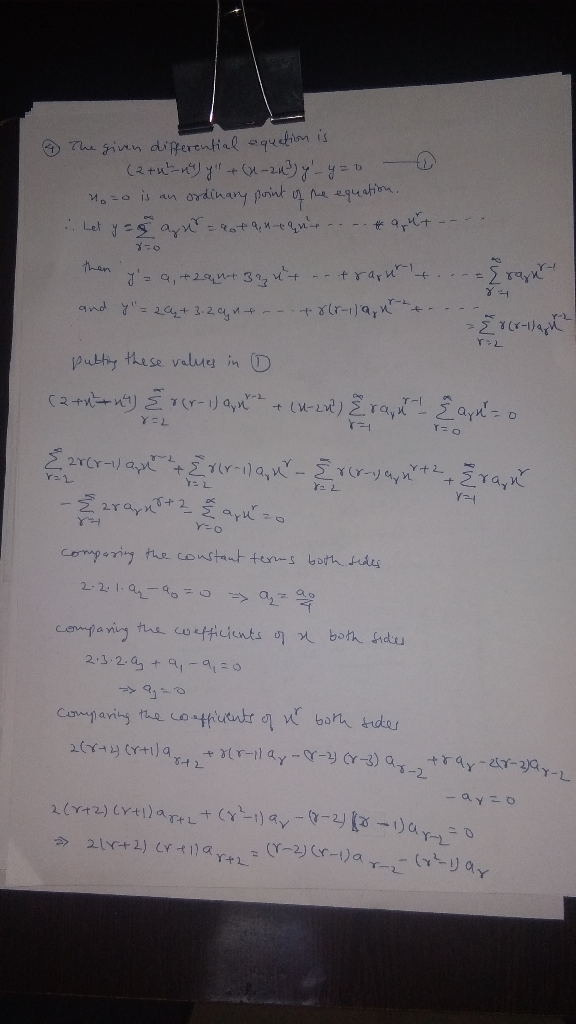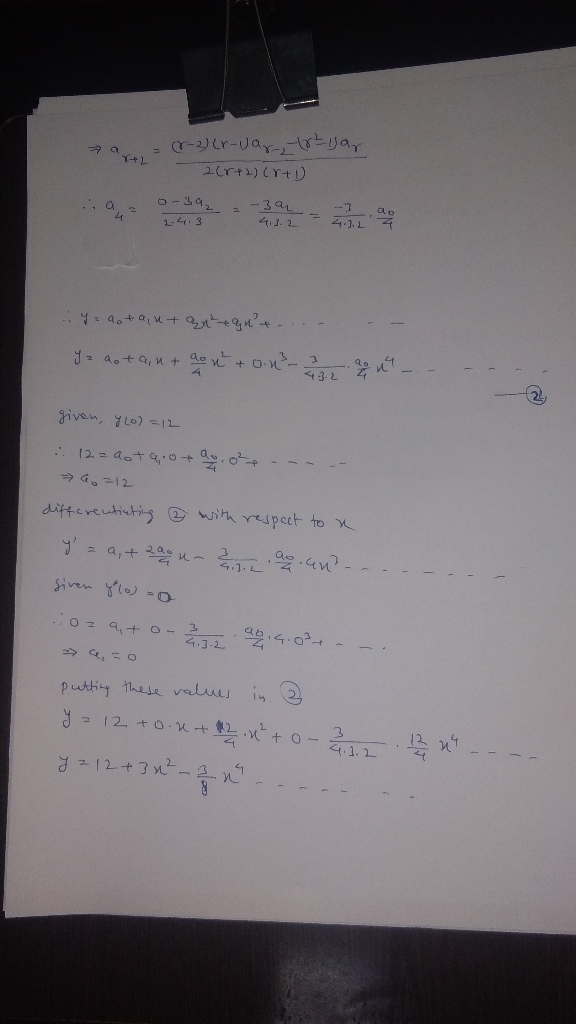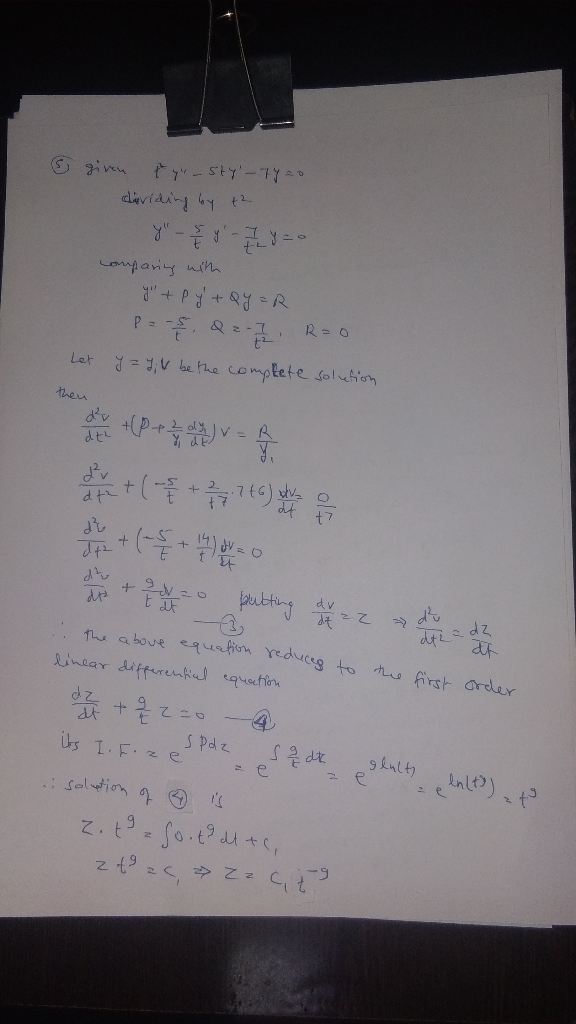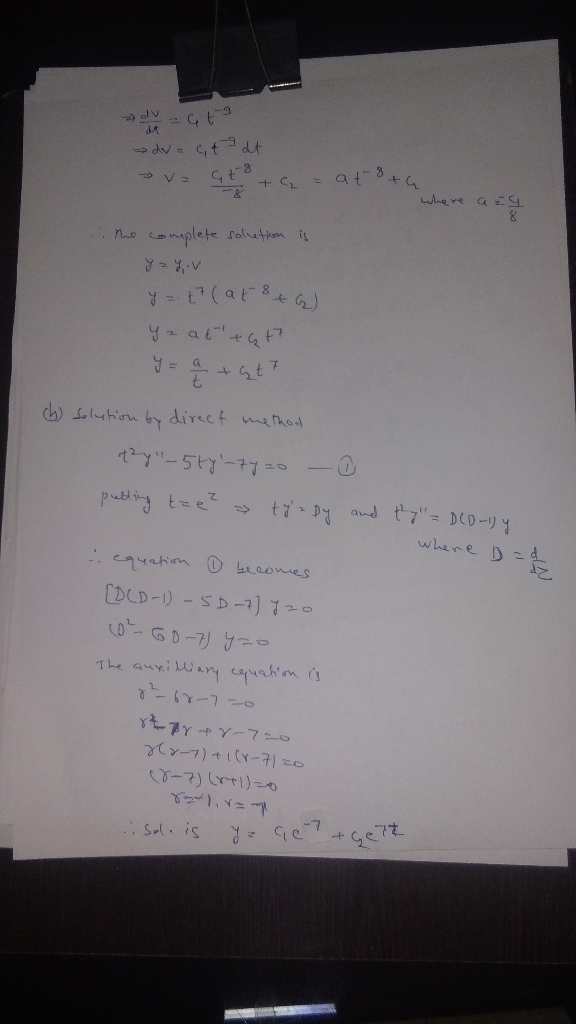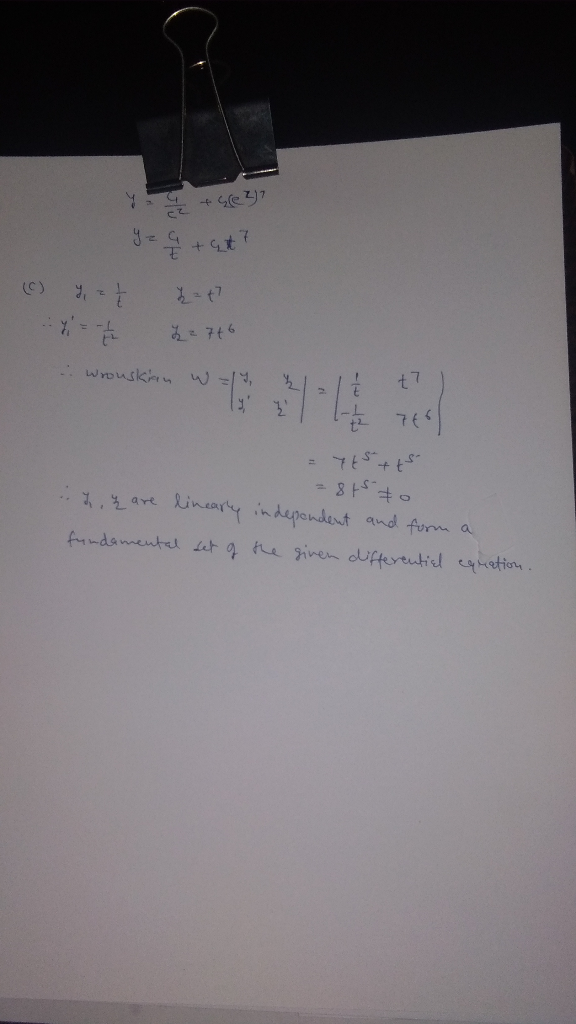Know the answer?
Your Answer:

#### Post as a guest

Your Name:

What's your source?

#### Earn Coin

Coins can be redeemed for fabulous gifts.

Not the answer you're looking for? Ask your own homework help question. Our experts will answer your question WITHIN MINUTES for Free.
Similar Homework Help Questions
• ### (6) (18 points) For the differential equation: Compute the recursion formula for the coefficients...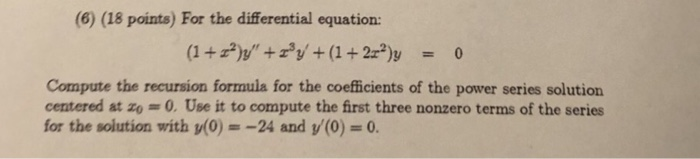(6) (18 points) For the differential equation: Compute the recursion formula for the coefficients of the power series solution centered at zo 0.Use it to compute the first three nonzero terms of the series for the solution with y(0) =-24 and y(0) = 0. (6) (18 points) For the differential equation: Compute the recursion formula for the coefficients of the power series solution centered at zo 0.Use it to compute the first three nonzero terms of the series for the...

• ### 3) Pol the diferential equation: (a) The point o -1 is an ordinary point. Compute the recursion formula for the coefficients of the power series solution centered at zo- -1 and use it to compute...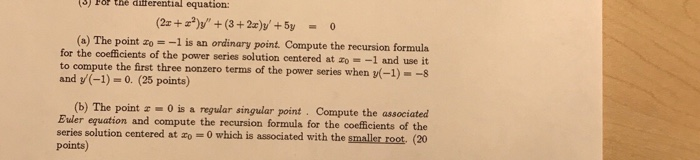3) Pol the diferential equation: (a) The point o -1 is an ordinary point. Compute the recursion formula for the coefficients of the power series solution centered at zo- -1 and use it to compute the first three nonzero terms of the power series when (-1)--s and y(-1)-0. (25 points) (b) The point 0 is a regular singular point Compute the associated Buler equation and compute the recursion formula for the coefficients of the series solution centered at o 0...

• ### It’s review question, I need this as soon as possible. Thank you 3) For thè diferential equation: (a) The point zo =-1 is an ordinary point. Compute the recursion formula for the coefficients of...It’s review question, I need this as soon as possible. Thank you3) For thè diferential equation: (a) The point zo =-1 is an ordinary point. Compute the recursion formula for the coefficients of the power series solution centered at zo- -1 and use it to compute the first three nonzero terms of the power series when -1)-s and v(-1)-0. (25 points) (b) The point z = 0 is a regular singular point . Compute the associated Euler equation and compute...

• ### B only It’s review question, I need this as soon as possible. Thank you 3) Pol the diferential equation: (a) The point o -1 is an ordinary point. Compute the recursion formula for the coeffici...B only It’s review question, I need this as soon as possible. Thank you 3) Pol the diferential equation: (a) The point o -1 is an ordinary point. Compute the recursion formula for the coefficients of the power series solution centered at zo- -1 and use it to compute the first three nonzero terms of the power series when (-1)--s and y(-1)-0. (25 points) (b) The point 0 is a regular singular point Compute the associated Buler equation and compute...

• ### 4. (a) Solve the differential equation (1-12)y"-2cy' + λ(A + 1)y 0 using power series centered at 0 , in which...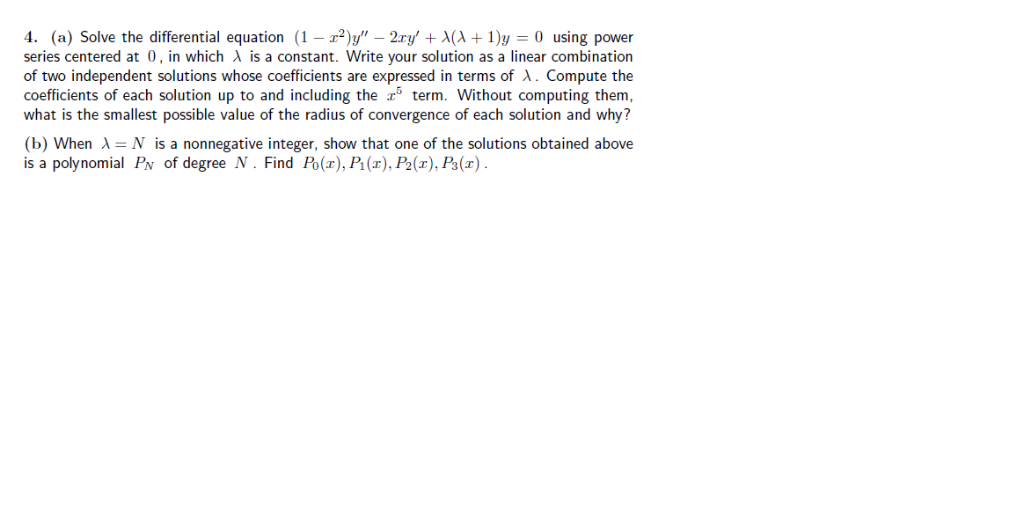4. (a) Solve the differential equation (1-12)y"-2cy' + λ(A + 1)y 0 using power series centered at 0 , in which λ is a constant. Write your solution as a linear combination of two independent solutions whose coefficients are expressed in terms of λ . Compute the coefficients of each solution up to and including the 5 term. Without computing them, what is the smallest possible value of the radius of convergence of each solution and why? (b) When λ...

• ### I need help with these! 3. (1 point) a) Compute the general solution of the differential equation y"5 12y" 0 b) Determine the test function Y (t) with the fewest terms to be used to obtain...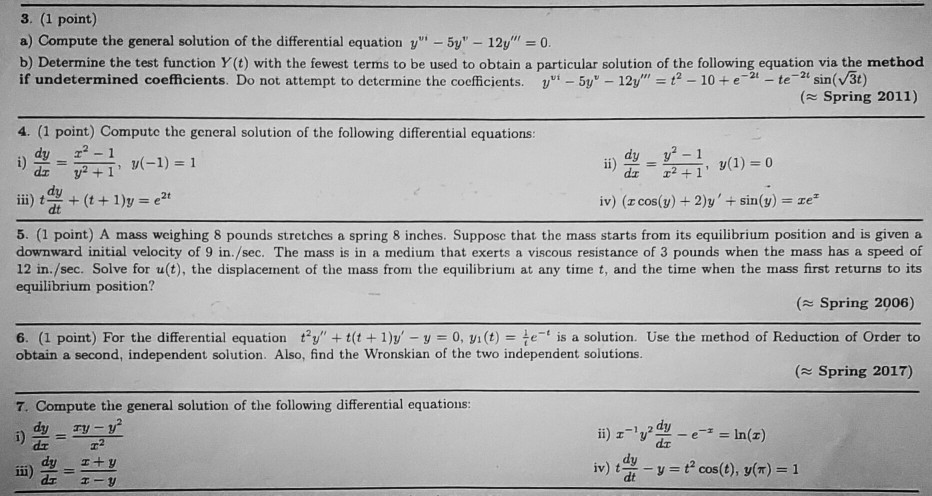I need help with these! 3. (1 point) a) Compute the general solution of the differential equation y"5 12y" 0 b) Determine the test function Y (t) with the fewest terms to be used to obtain a particular solution of the following equation via the method if undetermined coefficients. Do not attempt to determine the coefficients.5y 12y"2 10e-tesin(V3t) Spring 2011) 4. (1 point) Compute the general solution of the following differential equations dz dy dt ii)(1y iv) (z cos(y) +...

• ### 4. Find the first three nonzero terms in a power series expansion about xo = 0...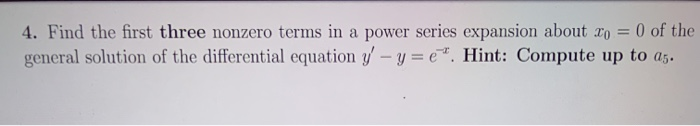4. Find the first three nonzero terms in a power series expansion about xo = 0 of the general solution of the differential equation y' - y=e". Hint: Compute up to 25.

• ### Use a power series centered about the ordinary point x0 = 0 to solve the differential equation (x − 4)y′′ − y′ + 12xy =...

Use a power series centered about the ordinary point x0 = 0 to solve the differential equation (x − 4)y′′ − y′ + 12xy = 0 Find the recurrence relation and at least the first four nonzero terms of each of the two linearly inde- pendent solutions (unless the series terminates sooner). What is the guaranteed radius of convergence?

• ### Give the first three nonzero (unsimplified) terms of the power series solution centered at co =...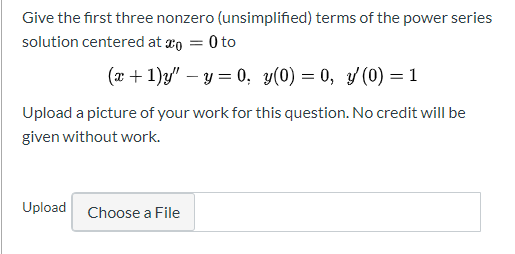Give the first three nonzero (unsimplified) terms of the power series solution centered at co = 0 to (x + 1)y" – y=0; y(0) = 0, y(0) = 1 Upload a picture of your work for this question. No credit will be given without work. Upload Choose a File

• ### Find the first four nonzero terms in a power series expansion about x = 0 for a general solution to the given differential equation.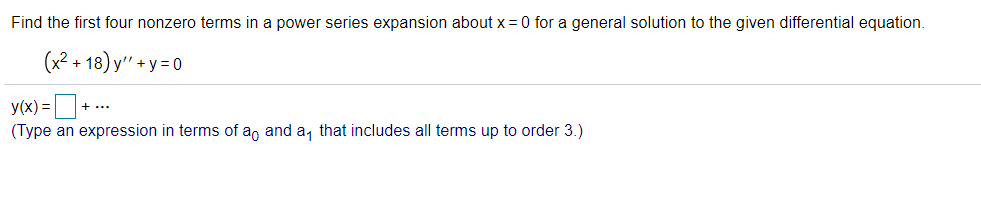Find the first four nonzero terms in a power series expansion about x = 0 for a general solution to the given differential equation.(x2 + 18)y'' + y = 0